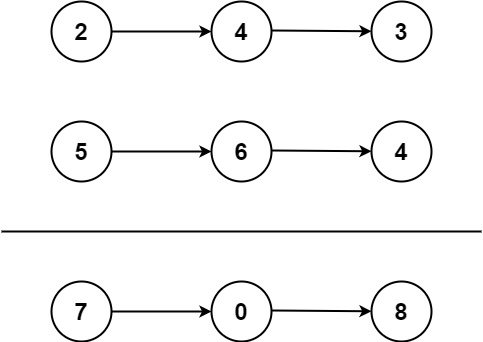Medium
You are given two non-empty linked lists representing two non-negative integers. The digits are stored in reverse order, and each of their nodes contains a single digit. Add the two numbers and return the sum as a linked list.
You may assume the two numbers do not contain any leading zero, except the number 0 itself.
Example 1:Input: l1 = [2,4,3], l2 = [5,6,4]
Output:
[7,0,8]
Explanation:
342 + 465 = 807.
Example 2:
Input: l1 = , l2 = 
Output:

Example 3:
Input: l1 = [9,9,9,9,9,9,9], l2 = [9,9,9,9]
Output:
[8,9,9,9,0,0,0,1]
Constraints:
• The number of nodes in each linked list is in the range `[1, 100]`.
• `0 <= Node.val <= 9`
• It is guaranteed that the list represents a number that does not have leading zeros.

### 解題

/**
* type ListNode struct {
* Val int
* Next *ListNode
* }
*/
func addTwoNumbers(l1 *ListNode, l2 *ListNode) *ListNode {
p1 := l1
p2 := l2
ans := &ListNode{}
now := ans
carry := 0
for p1 != nil || p2 != nil {
sum := carry
if p1 != nil {
sum += p1.Val
p1 = p1.Next
}
if p2 != nil {
sum += p2.Val
p2 = p2.Next
}
carry = sum / 10
now.Next = &ListNode{Val: sum % 10}
now = now.Next
}
if carry > 0 {
now.Next = &ListNode{Val: carry}
}
return ans.Next
}